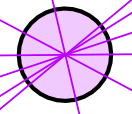# Lines of Symmetry of Plane Shapes

## Line of SymmetryHere my dog "Flame" has her face made perfectly symmetrical with a bit of photo magic. The white line down the center is the Line of Symmetry

## Folding Test

You can find if a shape has a Line of Symmetry by folding it.

When the folded part sits perfectly on top (all edges matching), then the fold line is a Line of Symmetry.

Here I have folded a rectangle one way, and it didn't work.So this is not a Line of Symmetry

But when I try it this way, it does work (the folded part sits perfectly on top, all edges matching):So this is a Line of Symmetry

## Triangles

A Triangle can have 3, or 1 or no lines of symmetry: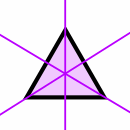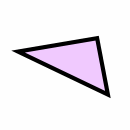Equilateral Triangle (all sides equal, all angles equal) Isosceles Triangle (two sides equal, two angles equal) Scalene Triangle (no sides equal, no angles equal) 3 Lines of Symmetry 1 Line of Symmetry No Lines of Symmetry

Different types of Quadrilaterals (a 4-sided plane shape):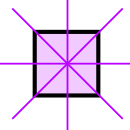Square (all sides equal, all angles 90°) Rectangle (opposite sides equal, all angles 90°) Irregular Quadrilateral 4 Lines of Symmetry 2 Lines of Symmetry No Lines of Symmetry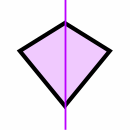Kite Rhombus (all sides equal length) 1 Line of Symmetry 2 Lines of Symmetry

## Regular Polygons

A regular polygon has all sides equal, and all angles equal:An Equilateral Triangle (3 sides) has 3 Lines of SymmetryA Square (4 sides) has 4 Lines of SymmetryA Regular Pentagon (5 sides) has 5 Lines of SymmetryA Regular Hexagon (6 sides) has 6 Lines of SymmetryA Regular Heptagon (7 sides) has 7 Lines of SymmetryA Regular Octagon (8 sides) has 8 Lines of Symmetry

And the pattern continues:

• A regular polygon of 9 sides has 9 Lines of Symmetry
• A regular polygon of 10 sides has 10 Lines of Symmetry
• ...
• A regular polygon of "n" sides has "n" Lines of Symmetry

## Circle

A line (drawn at any angle) that goes through its center is a Line of Symmetry.

So a Circle has infinite Lines of Symmetry.